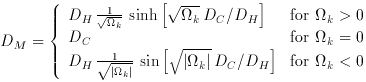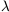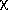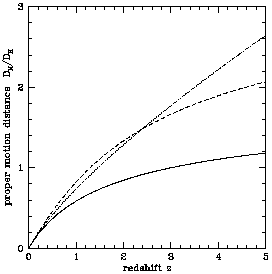### 5. COMOVING DISTANCE (TRANSVERSE)

The comoving distance between two events at the same redshift or distance but separated on the sky by some angleis DMand the transverse comoving distance DM (so-denoted for a reason explained below) is simply related to the line-of-sight comoving distance DC:(15)

where the trigonometric functions sinh and sin account for what is called ``the curvature of space.'' (Space curvature depends on the particular coordinate system chosen, so it is not intrinsic; a change of coordinates makes space flat; the only intrinsic curvature is space-time curvature, which is related to the local mass-energy density or really stress-energy tensor.) For= 0, there is an analytic solution to the equations(16)

(Weinberg 1972, p. 485; Peebles 1993, pp. 320-321). Some (eg., Weedman 1986, pp. 59-60) call this distance measure ``proper distance,'' which, though common usage, is bad style. (1)

(Although these notes follow the Peebles derivation, there is a qualitatively distinct method using what is known as the development angle, which increases as the Universe evolves. This method is preferred by relativists such as Misner, Thorne & Wheeler 1973, pp. 782-785).Figure 1. The dimensionless proper motion distance DM / DH. The three curves are for the three world models, Einstein-de Sitter (M,) = (1, 0), solid; low-density, (0.05, 0), dotted; and high lambda, (0.2, 0.8), dashed.

The comoving distance happens to be equivalent to the proper motion distance (hence the name DM), defined as the ratio of the actual transverse velocity (in distance over time) of an object to its proper motion (in radians per unit time) (Weinberg 1972, pp. 423-424). The proper motion distance is plotted in Figure 1. Proper motion distance is used, for example, in computing radio jet velocities from knot motion.

1 The word ``proper'' has a specific use in relativity. The proper time between two nearby events is the time delay between the events in the frame in which they take place at the same location, and the proper distance between two nearby events is the distance between them in the frame in which they happen at the same time. It is the distance measured by a ruler at the time of observation. The transverse comoving distance DM is not a proper distance - it is a proper distance divided by a ratio of scale factors. Back.